# 跳躍表資料結構與演算法分析

1. 通過搜尋引用，可以保證從任意元素開始，搜尋到在列表中間隔為k的元素的任意期望時間是O(logk)
2. 實現線性表的常規操作（例如_將元素插入到列表第k個元素後面_）

# 預覽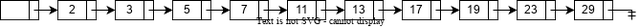Figure.2表示一個連結串列，在該連結串列中，每個一個節點就有一個附加的指標指向它在表中的前兩個位置上的節點。正因為這個前向指標，在最壞情況下最多考查⌈N/2⌉+1個節點。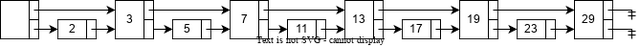Figure.2 Linked List with fingers to the 2nd forward elements

Figure.3將這種想法擴充套件，每個序數是4的倍數的節點都有一個指標指向下一個序數為4的倍數的節點。只有⌈N/4⌉+2個節點被考查。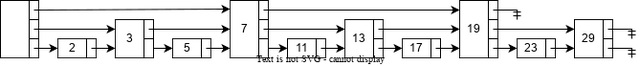Figure.3 Linked List with fingers to the 4th forward elements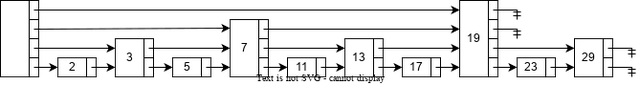Figure.4 Linked List with fingers to the 2ith forward elements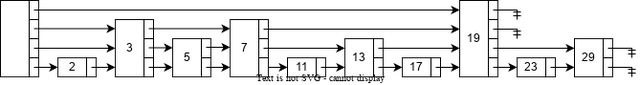Figure.5 Skip List

# 資料結構

1. 所有元素在跳躍表中都是由一個節點表示。
2. 每個節點都有一個高度或級別，有時候也可以稱之為階（step），節點的級別是一個與元素總數無關的隨機數。規定NULL的級別是∞。
3. 每個級別為k的節點都有k個前向指標，且第i個前向指標指向下一個級別為i或更高的節點。
4. 每個節點的級別都不會超過一個明確的常量MaxLevel。整個跳躍表的級別是所有節點的級別的最高值。如果跳躍表是空的，那麼跳躍表的級別就是1。

``````#define SKIP_LIST_KEY_TYPE     int
#define SKIP_LIST_VALUE_TYPE   int
#define SKIP_LIST_MAX_LEVEL    32
#define SKIP_LIST_P            0.5

struct Node {
SKIP_LIST_KEY_TYPE    key;
SKIP_LIST_VALUE_TYPE  value;
struct Node          *forwards[]; // flexible array member
};

struct SkipList {
int          level;
};

struct Node *CreateNode(int level) {
struct Node *node;
assert(level > 0);
node = malloc(sizeof(struct Node) + sizeof(struct Node *) * level);
return node;
}

struct SkipList *CreateSkipList() {
struct SkipList *list;
int              i;

list = malloc(sizeof(struct SkipList));
for (i = 0; i < SKIP_LIST_MAX_LEVEL; i++) {
}
list->level = 1;

return list;
}

``````

# 演算法

## 搜尋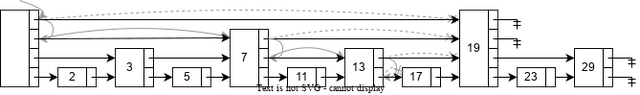Figure.6 A search path to find element 17 in Skip List

``````struct Node *SkipListSearch(struct SkipList *list, SKIP_LIST_KEY_TYPE target) {
struct Node *current;
int          i;

for (i = list->level - 1; i >= 0; i--) {
while (current->forwards[i] && current->forwards[i]->key < target) {
current = current->forwards[i];
}
}

current = current->forwards;
if (current->key == target) {
return current;
} else {
return NULL;
}
}

``````

## 插入和刪除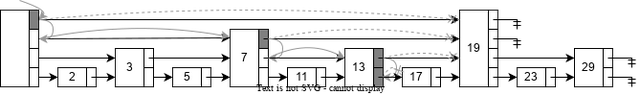Figure.7 Search path for inserting element 16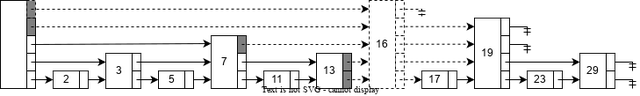Figure.8 Insert element 16 and adjust forward pointers

``````struct Node *SkipListInsert(struct SkipList *list, SKIP_LIST_KEY_TYPE key, SKIP_LIST_VALUE_TYPE value) {
struct Node *update[SKIP_LIST_MAX_LEVEL];
struct Node *current;
int          i;
int          level;

for (i = list->level - 1; i >= 0; i--) {
while (current->forwards[i] && current->forwards[i]->key < target) {
current = current->forwards[i];
}
update[i] = current;
}

current = current->forwards;
if (current->key == target) {
current->value = value;
return current;
}

level = SkipListRandomLevel();
if (level > list->level) {
for (i = list->level; i < level; i++) {
}
}

current = CreateNode(level);
current->key = key;
current->value = value;

for (i = 0; i < level; i++) {
current->forwards[i] = update[i]->forwards[i];
update[i]->forwards[i] = current;
}

return current;
}

``````

Figure.9和Figure.10展示了在跳躍表中刪除元素19的過程。首先，在Figure.9中執行與搜尋相同的查詢過程，在每個級別遍歷到的最後一個元素在對應層級的前向指標被標記為灰色，表示稍後將會對齊進行調整。接下來在Figure.10中，首先通過調整前向指標將元素19對應的節點從跳躍表中解除安裝，因為元素19對應的節點是級別最高的節點，因此將其從跳躍表中移除後需要調整跳躍表的級別。Figure.10中所有虛線部分都表示調整後的效果。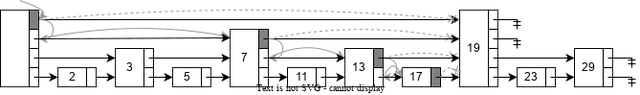Figure.9 Search path for deleting element 19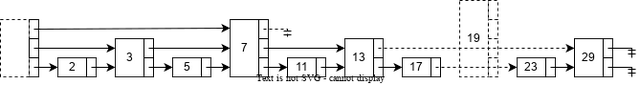Figure.10 Delete element 19 and adjust forward pointers

``````struct Node *SkipListDelete(struct SkipList *list, SKIP_LIST_KEY_TYPE key) {
struct Node *update[SKIP_LIST_MAX_LEVEL];
struct Node *current;
int          i;

for (i = list->level - 1; i >= 0; i--) {
while (current->forwards[i] && current->forwards[i]->key < key) {
current = current->forwards[i];
}
update[i] = current;
}

current = current->forwards;
if (current && current->key == key) {
for (i = 0; i < list->level; i++) {
if (update[i]->forwards[i] == current) {
update[i]->forwards[i] = current->forwards[i];
} else {
break;
}
}

while (list->level > 1 && list->head->forwards[list->level - 1] == NULL) {
list->level--;
}
}

return current;
}

``````

## 生成隨機級別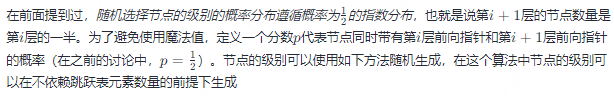``````int SkipListRandomLevel() {
int level;
level = 1;
while (random() < RAND_MAX * SKIP_LIST_P && level <= SKIP_LIST_MAX_LEVEL) {
level++;
}
return level;
}

``````

1 2 3 4 5 6 7 8
2147540777 1073690199 536842769 268443025 134218607 67116853 33563644 16774262
9 10 11 12 13 14 15 16
8387857 4193114 2098160 1049903 523316 262056 131455 65943
17 18 19 20 21 22 23 24
32611 16396 8227 4053 2046 1036 492 249
25 26 27 28 29 30 31 32
121 55 34 16 7 9 2 1

## MaxLevel的選擇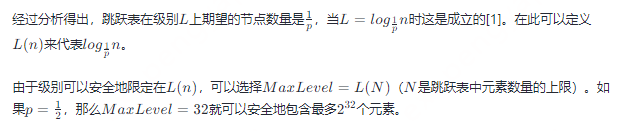# 分析

## 空間複雜度分析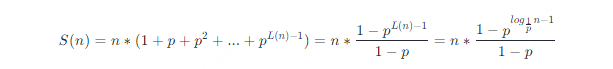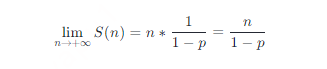## 時間複雜度分析

### 非形式化分析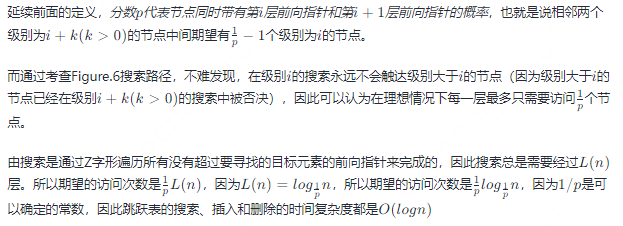### 形式化分析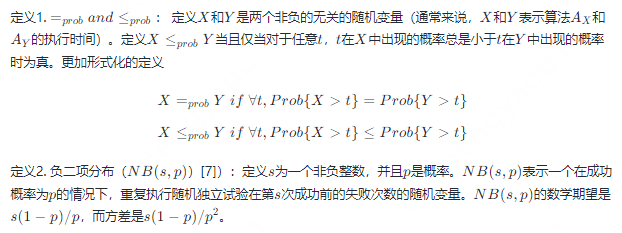C(n)≤probC=prob(L(n)−1)+NB(L(n)−1,p)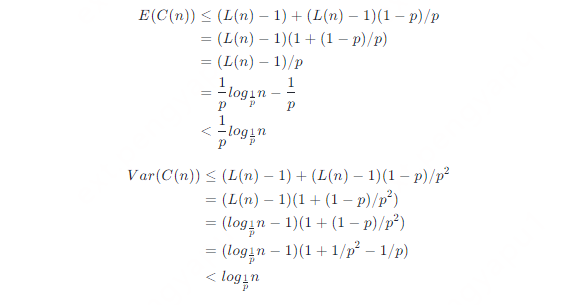### P的選擇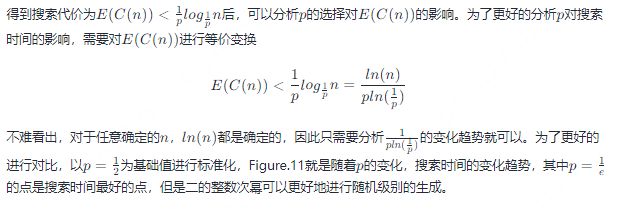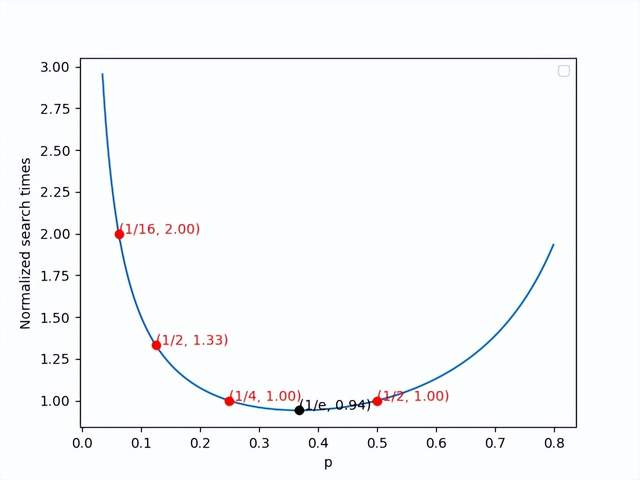Figure.11 Normalized search times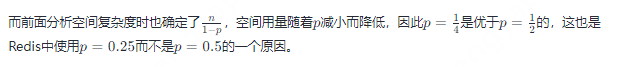# 擴充套件

## 快速隨機訪問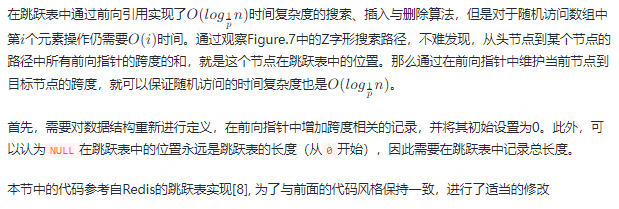``````#define SKIP_LIST_KEY_TYPE     int
#define SKIP_LIST_VALUE_TYPE   int
#define SKIP_LIST_MAX_LEVEL    32
#define SKIP_LIST_P            0.5

struct Node; // forward definition

struct Forward {
struct Node *forward;
int          span;
}

struct Node {
SKIP_LIST_KEY_TYPE    key;
SKIP_LIST_VALUE_TYPE  value;
struct Forward        forwards[]; // flexible array member
};

struct SkipList {
int          level;
int          length;
};

struct Node *CreateNode(int level) {
struct Node *node;
assert(level > 0);
node = malloc(sizeof(struct Node) + sizeof(struct Forward) * level);
return node;
}

struct SkipList *CreateSkipList() {
struct SkipList *list;
int              i;

list = malloc(sizeof(struct SkipList));
for (i = 0; i < SKIP_LIST_MAX_LEVEL; i++) {
}

list->level = 1;

return list;
}

``````

``````struct Node *SkipListInsert(struct SkipList *list, SKIP_LIST_KEY_TYPE key, SKIP_LIST_VALUE_TYPE value) {
struct Node *update[SKIP_LIST_MAX_LEVEL];
struct Node *current;
int          indices[SKIP_LIST_MAX_LEVEL];
int          i;
int          level;

for (i = list->level - 1; i >= 0; i--) {
if (i == list->level - 1) {
indices[i] = 0;
} else {
indices[i] = indices[i + 1];
}

while (current->forwards[i].forward && current->forwards[i].forward->key < target) {
indices[i] += current->forwards[i].span;
current = current->forwards[i].forward;
}
update[i] = current;
}

current = current->forwards.forward;
if (current->key == target) {
current->value = value;
return current;
}

level = SkipListRandomLevel();
if (level > list->level) {
for (i = list->level; i < level; i++) {
indices[i] = 0;
update[i]->forwards[i].span = list->length;
}
}

current = CreateNode(level);
current->key = key;
current->value = value;

for (i = 0; i < level; i++) {
current->forwards[i].forward = update[i]->forwards[i].forward;
update[i]->forwards[i].forward = current;
current->forwards[i].span = update[i]->forwards[i].span - (indices - indices[i]);
update[i]->forwards[i].span = (indices - indices[i]) + 1;
}

list.length++;

return current;
}

``````
``````struct Node *SkipListDelete(struct SkipList *list, SKIP_LIST_KEY_TYPE key) {
struct Node *update[SKIP_LIST_MAX_LEVEL];
struct Node *current;
int          i;

for (i = list->level - 1; i >= 0; i--) {
while (current->forwards[i].forward && current->forwards[i].forward->key < key) {
current = current->forwards[i].forward;
}
update[i] = current;
}

current = current->forwards.forward;
if (current && current->key == key) {
for (i = 0; i < list->level; i++) {
if (update[i]->forwards[i].forward == current) {
update[i]->forwards[i].forward = current->forwards[i];
update[i]->forwards[i].span += current->forwards[i].span - 1;
} else {
break;
}
}

while (list->level > 1 && list->head->forwards[list->level - 1] == NULL) {
list->level--;
}
}

return current;
}

``````

# 參考文獻

1. Pugh, W. (1989). A skip list cookbook. Tech. Rep. CS-TR-2286.1, Dept. of Computer Science, Univ. of Maryland, College Park, MD [July 1989]
2. Pugh, W. (1989). Skip lists: A probabilistic alternative to balanced trees. Lecture Notes in Computer Science, 437–449. https://doi.org/10.1007/3-540-51542-9_36
3. Weiss, M. A. (1996).Data Structures and Algorithm Analysis in C (2nd Edition)(2nd ed.). Pearson.
4. Aragon, Cecilia & Seidel, Raimund. (1989). Randomized Search Trees. 540-545. 10.1109/SFCS.1989.63531.
5. Wikipedia contributors. (2022b, November 22).Finger search. Wikipedia. https://en.wikipedia.org/wiki/Finger_search